# How to square root in Excel: SQRT function and other ways

The tutorial shows how to do square root in Excel as well as how to calculate Nth root of any value.

Squaring a number and taking a square root are very common operations in mathematics. But how do you do square root in Excel? Either by using the SQRT function or by raising a number to the power of 1/2. The following examples show full details.

## How to square root in Excel using SQRT function

The easiest way to do square root in Excel is by using the function specially designed for this:

SQRT(number)

Where number is the number or reference to the cell containing the number for which you want to find the square root.

For example, to get a square root of 225, you use this formula: `=SQRT(225)`

To calculate square root of a number in A2, use this one: `=SQRT(A2)`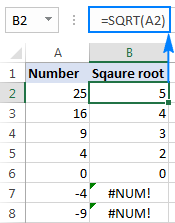If a number is negative, like in rows 7 and 8 in the screenshot above, the Excel SQRT function returns the #NUM! error. It happens because the square root of a negative number does not exist among the set of real numbers. Why's that? Since there is no way to square a number and get a negative result.

In case you wish to take a square root of a negative number as if it were a positive number, wrap the source number in the ABS function, which returns the absolute value of a number ignoring the sign:

`=SQRT(ABS(A2))`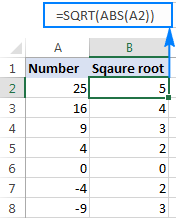## How to do square root in Excel using a calculation

When calculating by hand, you write square root by using the radical symbol (√). Though, it's not possible to type that traditional square root symbol in Excel, there is a way to find square root without any function. For this, you use the caret character (^), which is located above the number 6 on most keyboards.

In Microsoft Excel, the caret symbol (^) acts as the exponent, or power, operator. For example, to square the number 5, i.e. raise 5 to the power of 2, you type =5^2 in a cell, which is equivalent to 52.

To get a square root, use the caret with (1/2) or 0.5 as the exponent:

number^(1/2)

or

number^0.5

For example, to get the square root of 25, you type `=25^(1/2)` or `=25^0.5` in a cell.

To find square root of a number in A2, you type: `=A2^(1/2)` or `=A2^0.5`

As shown in the screenshot below, the Excel SQRT function and the exponent formula yield identical results: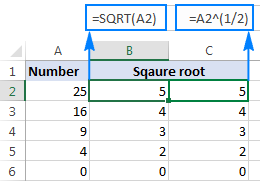This square root expression can also be used as part of bigger formulas. For instance, the following IF statement tells Excel to calculate a square root on condition: get a square root if A2 contains a number, but return an empty string (blank cell) if A2 is a text value or blank:

`=IF(ISNUMBER(A2), A2^(1/2), "")`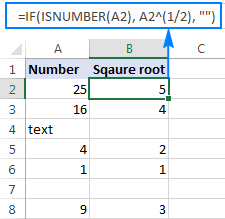#### Why is an exponent of 1/2 the same as square root?

For starters, what do we call a square root? It is nothing else but a number that, when multiplied by itself, gives the original number. For example, the square root of 25 is 5 because 5x5=25. That is crystal clear, isn't it?

Well, multiplying 251/2 by itself also gives 25:

25½ x 25½ = 25(½+½) = 25(1) = 25

Said another way:

25 x √25 = 25

And:

25½ x 25½ = 25

So, 25½ is equivalent to √25.

## How to find square root with POWER function

The POWER function is just another way to perform the above calculation, i.e. raise a number to the power of 1/2.

The syntax of the Excel POWER function is as follows:

POWER(number, power)

As you can easily guess, to get a square root, you supply 1/2 to the power argument. For example:

`=POWER(A2, 1/2)`

As shown in the screenshot below, all three square root formulas produce identical result, which one to use is a matter of your personal preference: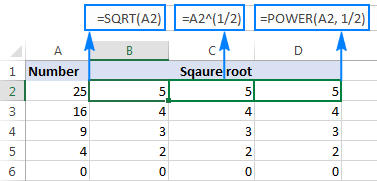## How to calculate Nth root in Excel

The exponent formula discussed a few paragraphs above is not limited to finding only a square root. The same techniques can be used to get any nth root - just type the desired root in the denominator of a fraction after the caret character:

number^(1/n)

Where number is the number you'd like to find the root of and n is the root.

For example:

• The cube root of 64 would be written as: =64^(1/3)
• To get the 4th root of 16, you type: =16^(1/4)
• To find the 5th root of a number in cell A2, you type: =A2^(1/5)

Instead of fractions, you can use decimal numbers in exponents, of course if the decimal form of the fraction has a reasonable number of decimal places. For instance, to calculate the 4th root of 16, you can go with either =16^(1/4) or =16^0.25.

Please notice that fractional exponents should always be enclosed in parenthesis to ensure the proper order of operations in your square root formula - first division (the forward slash (/) is the division operator in Excel), and then raising to the power.

The same results can be achieved by using the POWER function:

• The cube root of 64: =POWER(64, 1/3)
• The 4th root of 16: =POWER(16, 1/4)
• The 5th root of a number in cell A2: =POWER(A2, 1/5)

In your real-life worksheets, you can type the roots in separate cells, and reference those cells in your formulas. For example, here's how you find the root input in B2 of the number in A3:

`=\$A3^(1/B\$2)`

The screenshot below shows the results rounded to 2 decimal places: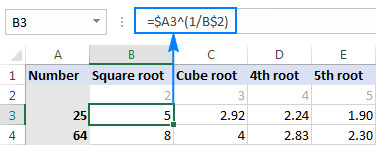Tip. To perform multiple calculations with a single formula like in the above example, fix a column and/or row reference where appropriate by using the dollar sign (\$). For more information, please see Why use dollar sign in Excel formulas.

This is how you can do square root in Excel. I thank you for reading and hope to see you on our blog next week!

## You may also be interested in

### 4 responses to "How to square root in Excel: SQRT function and other ways"

1.kantharaj says:

Hi Svetlana, Please let me know how VBA coding for Index and match function from different sheet. Thanks!

2.Syed Ali says:

Dear Svetlana

I have a situation , where I need to calculate the calls made between certain time by an helpdesk agent.

Example; Agent X make some calls during his 8 hours duty time from 8:am to 4 Pm.

I want to the formula to calculate calls made between 11: am to 1pm.

really appreciate

3.Mark says:

Can you combine functions like power & sqrt, if so can you provide an example. The reason i am asking I and trying to find the Slope Factor of a roof.
I use this formula in paper and would like to use Excel.

5/12, squared, +1, sqrt

4.DARSHAN GANGARAM RESHIM says:

I want answer of 25th SQRT 5 Power 3 in Excel single column